# R S Aggarwal Solutions for Class 10 Maths Chapter 15 Perimeter and Area of Plane Figures Exercise 15B

R S Aggarwal Solutions are the best study material for the students to score good marks in the board exams. Get your Class 10 Maths Chapter 15 Perimeter and Area of Plane Figures exercise 15B solutions here. This exercise deals with the area and perimeter of plane figures with the help of some important concepts and formulas. Students can get detailed explanations of all the questions here. Click on the link below to get your PDF now.

## Download PDF of R S Aggarwal Solutions for Class 10 Maths Chapter 15 Perimeter and Area of Plane Figures Exercise 15B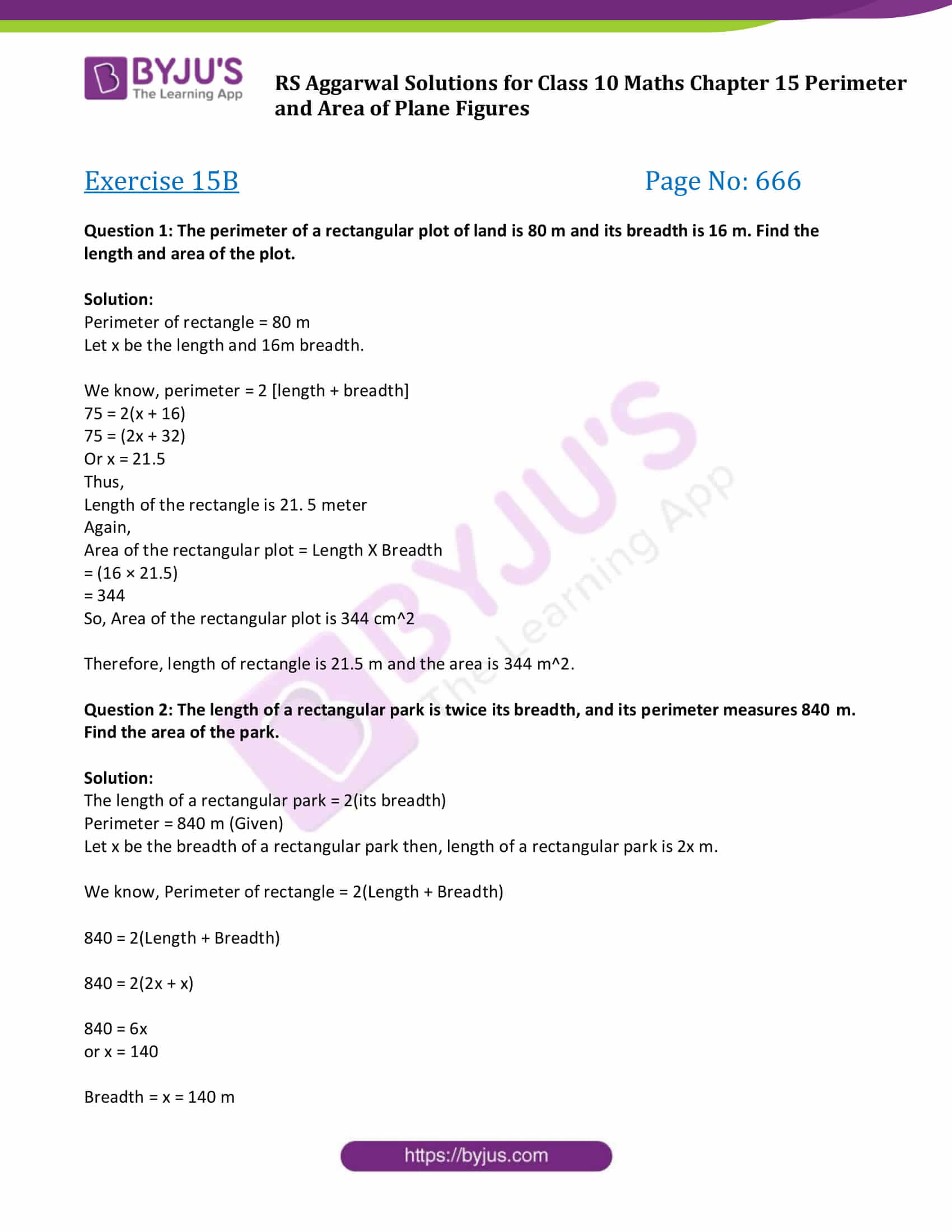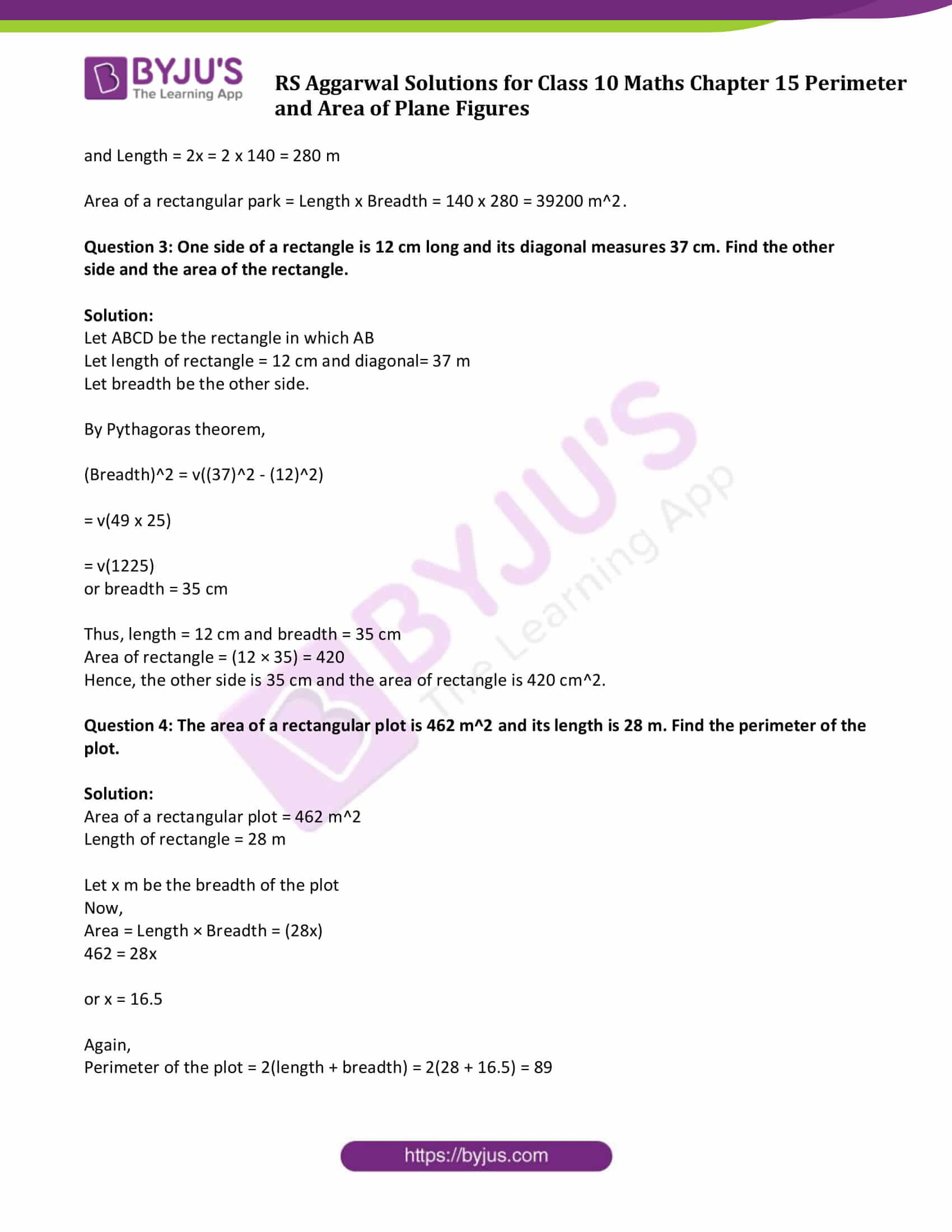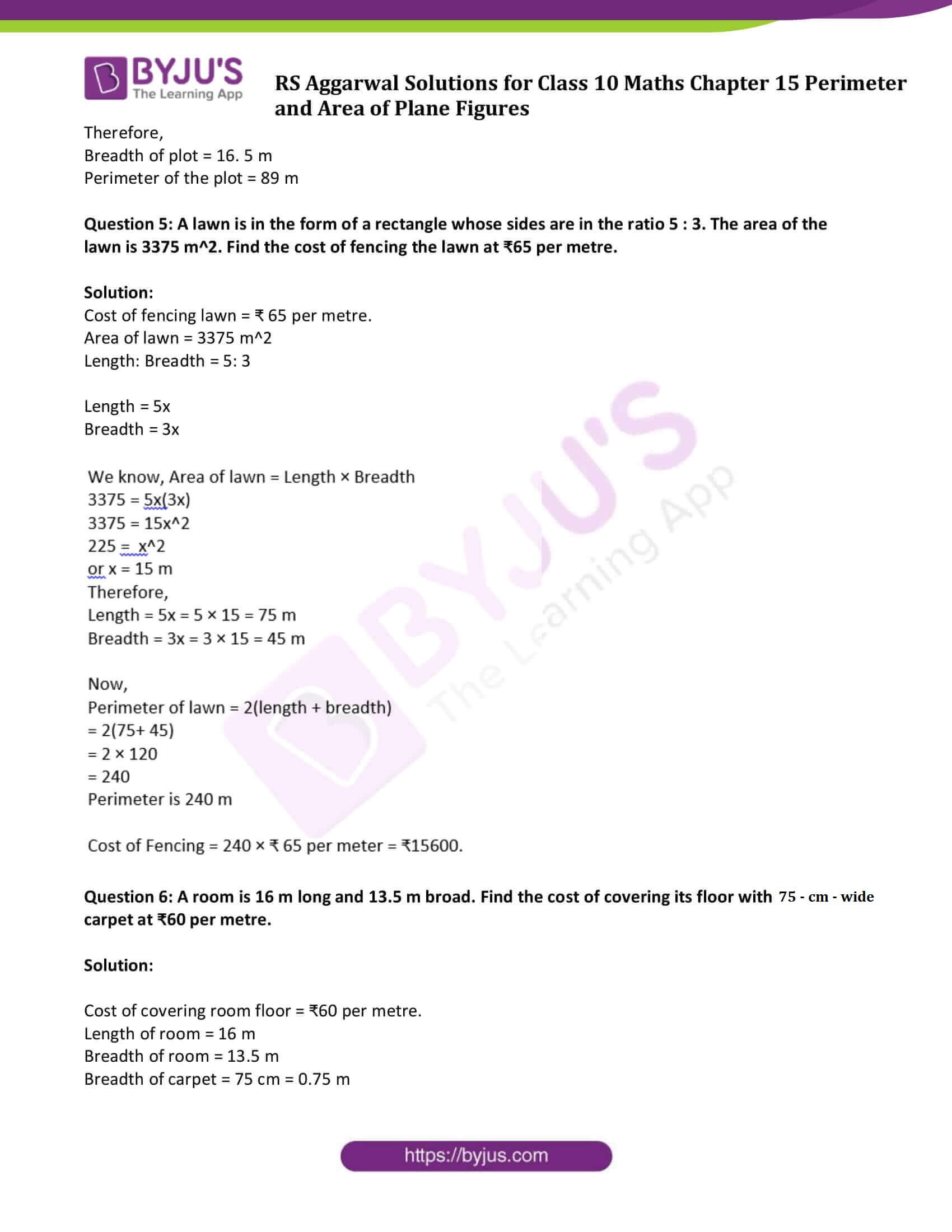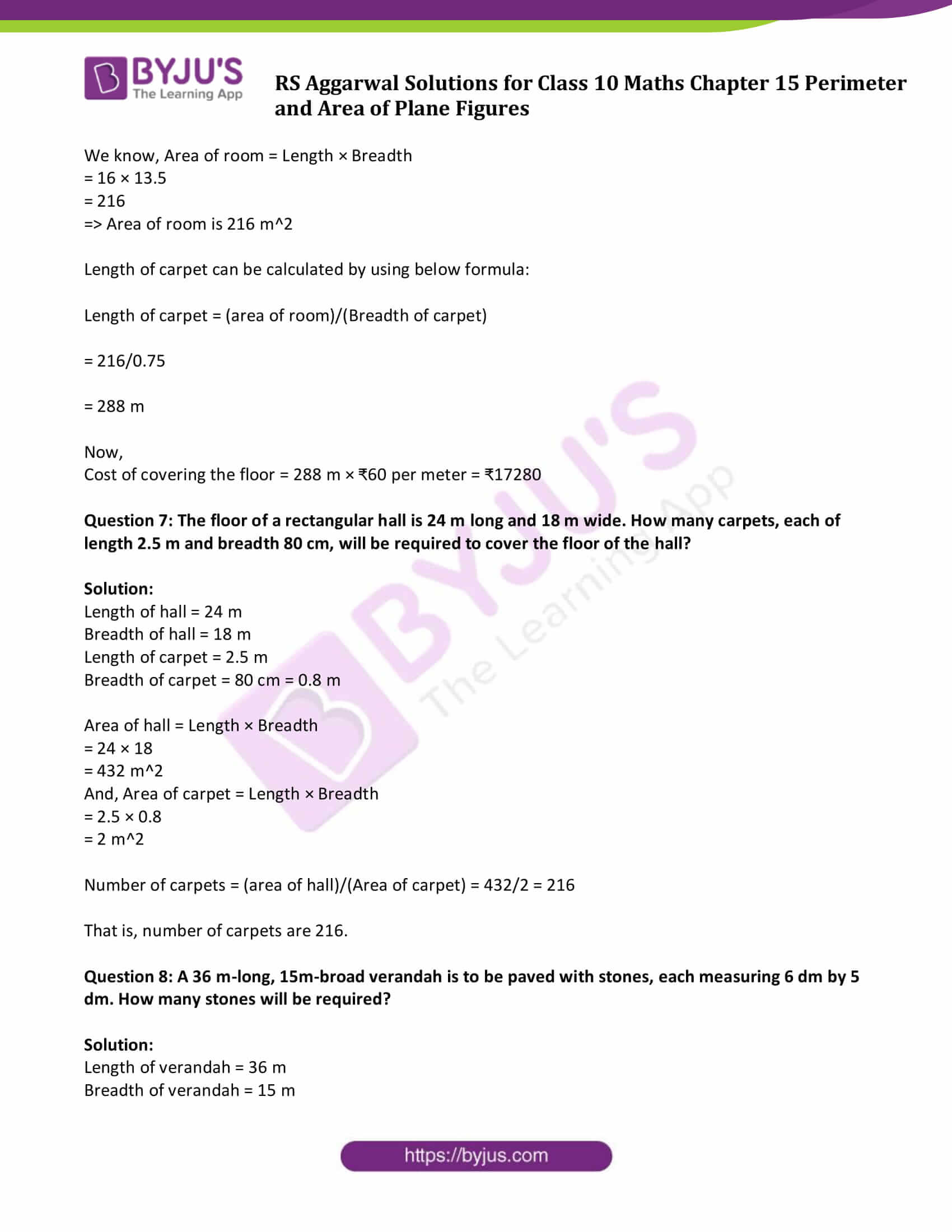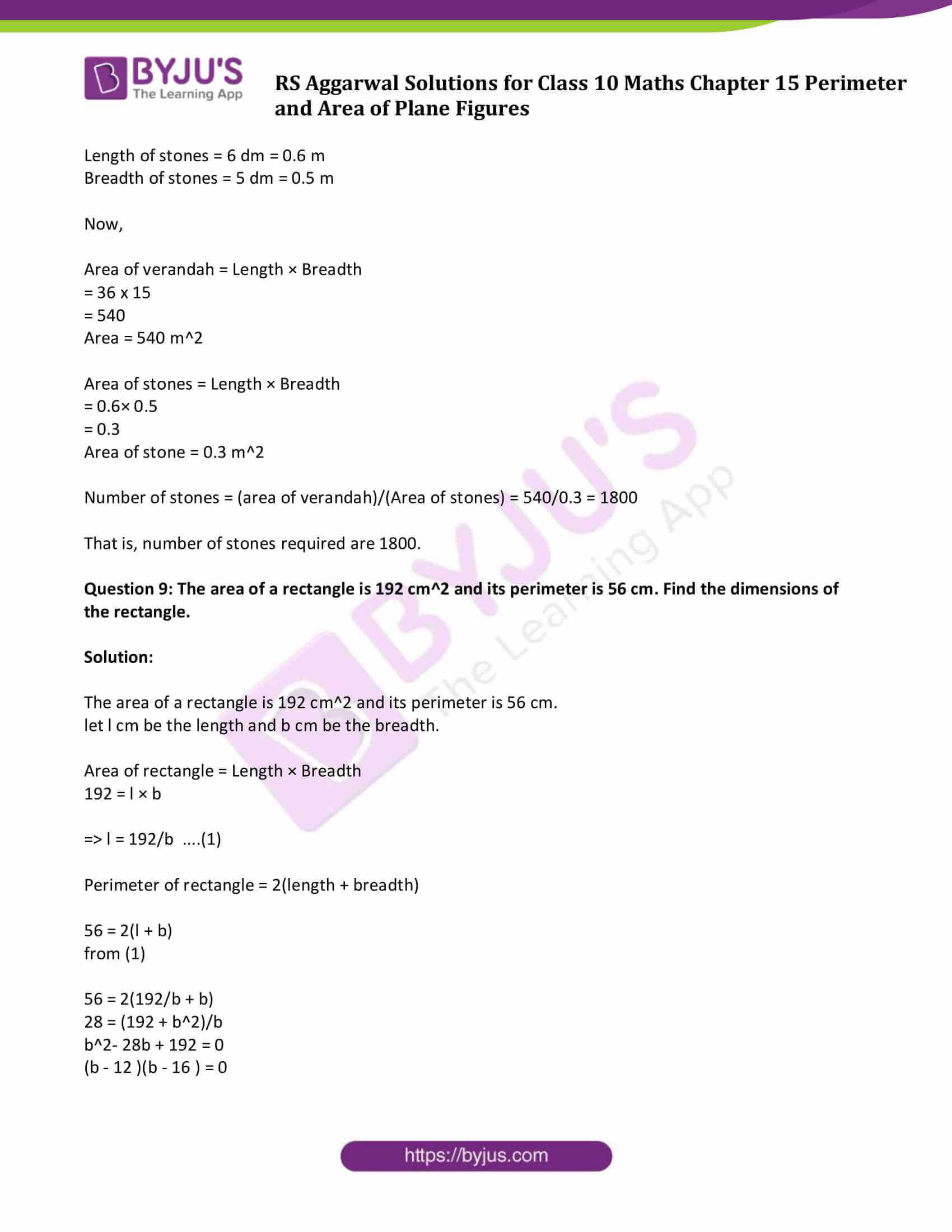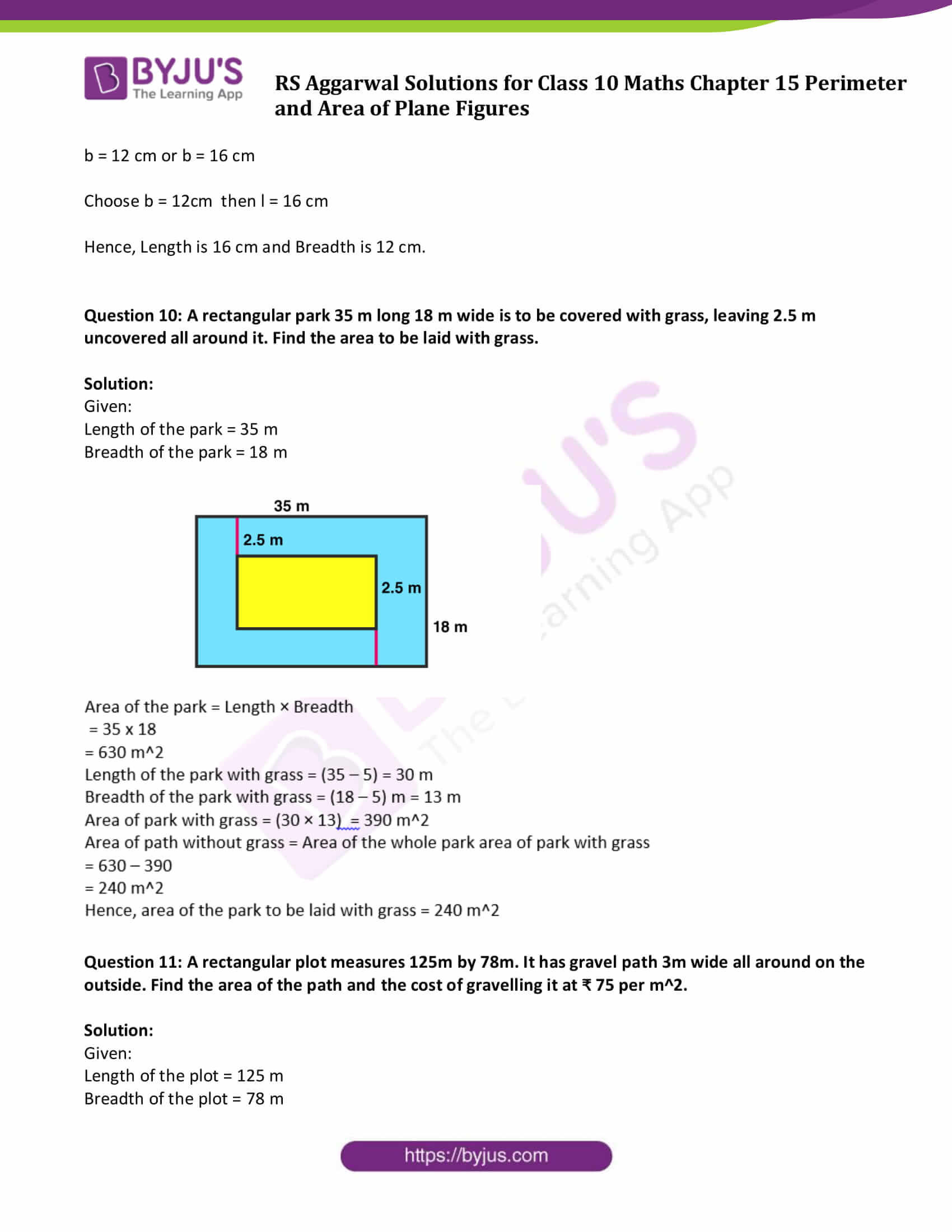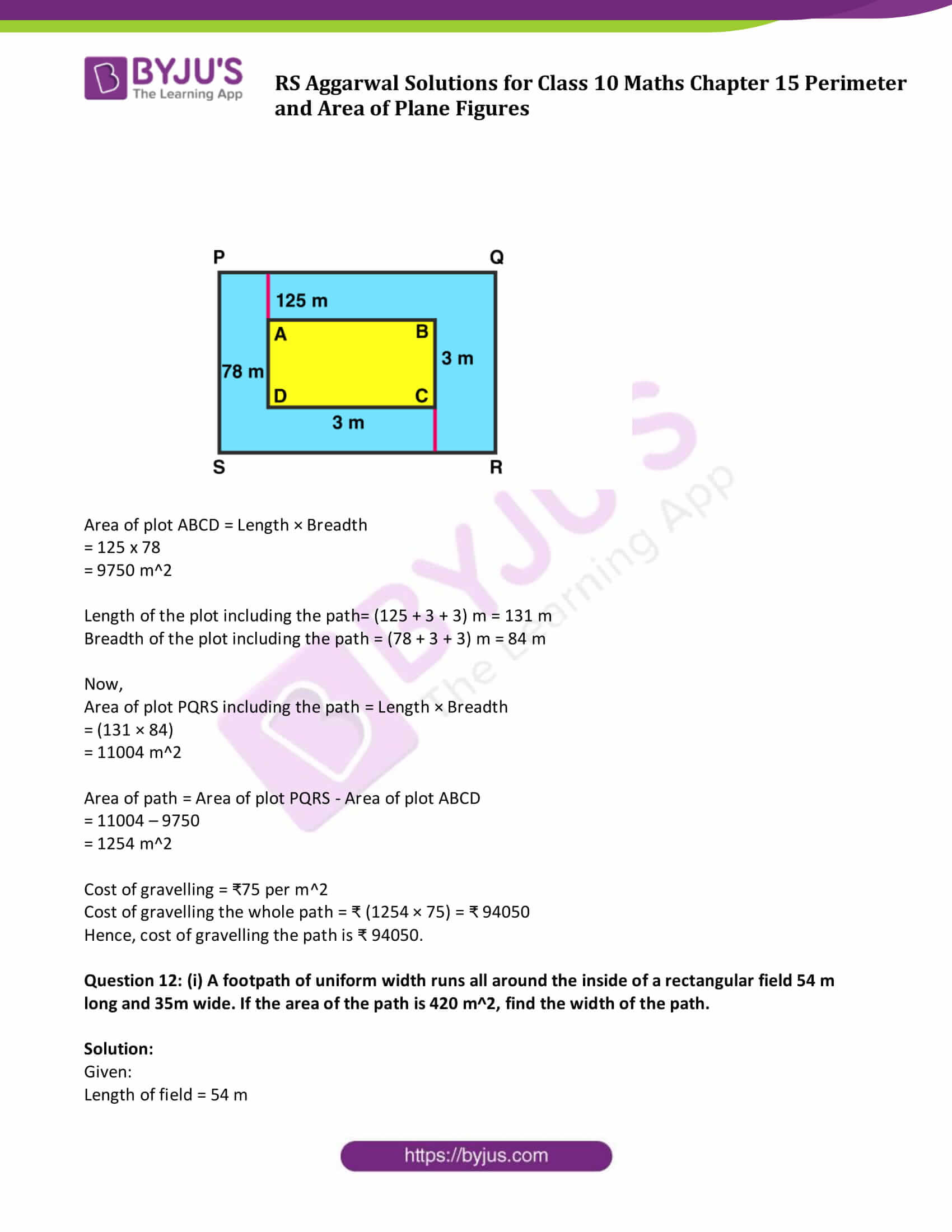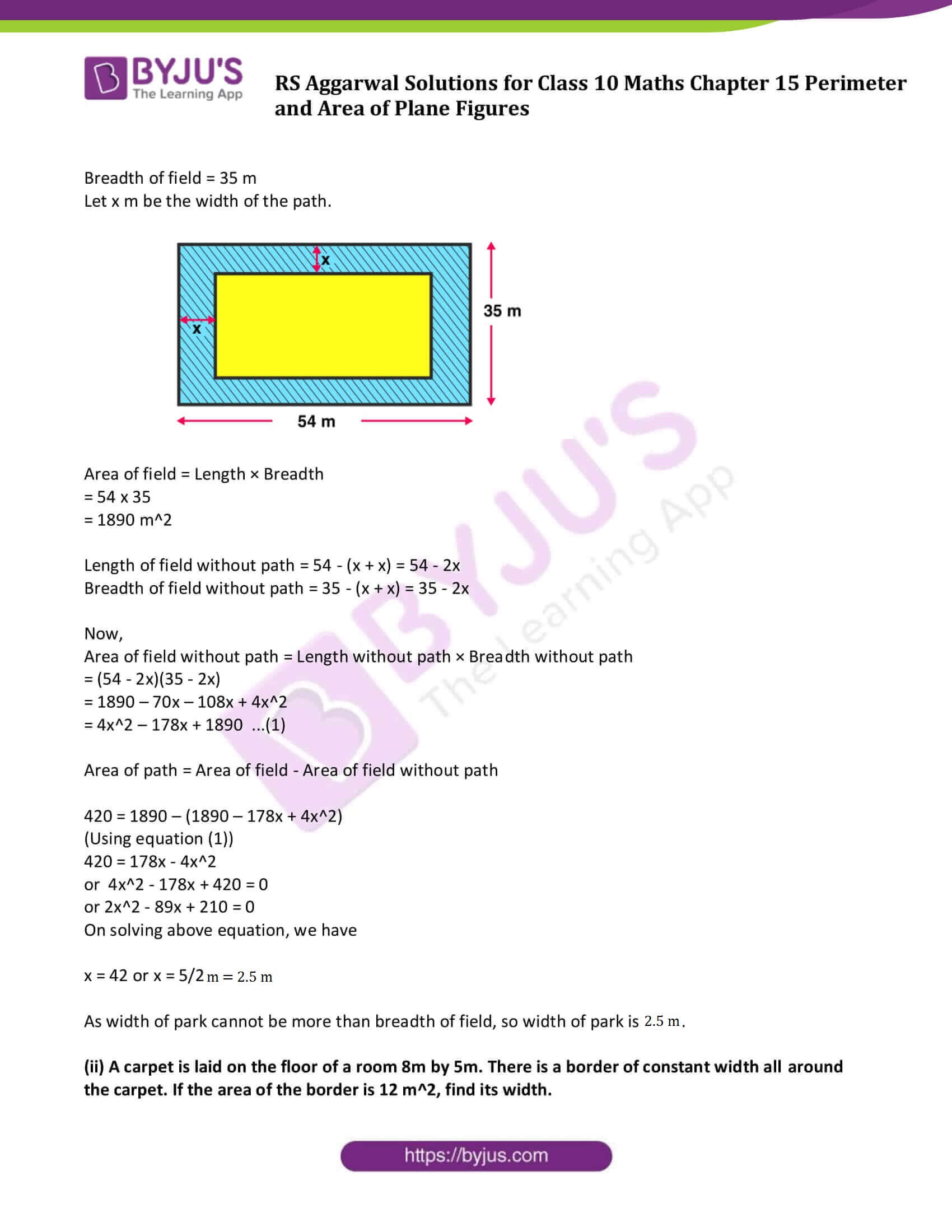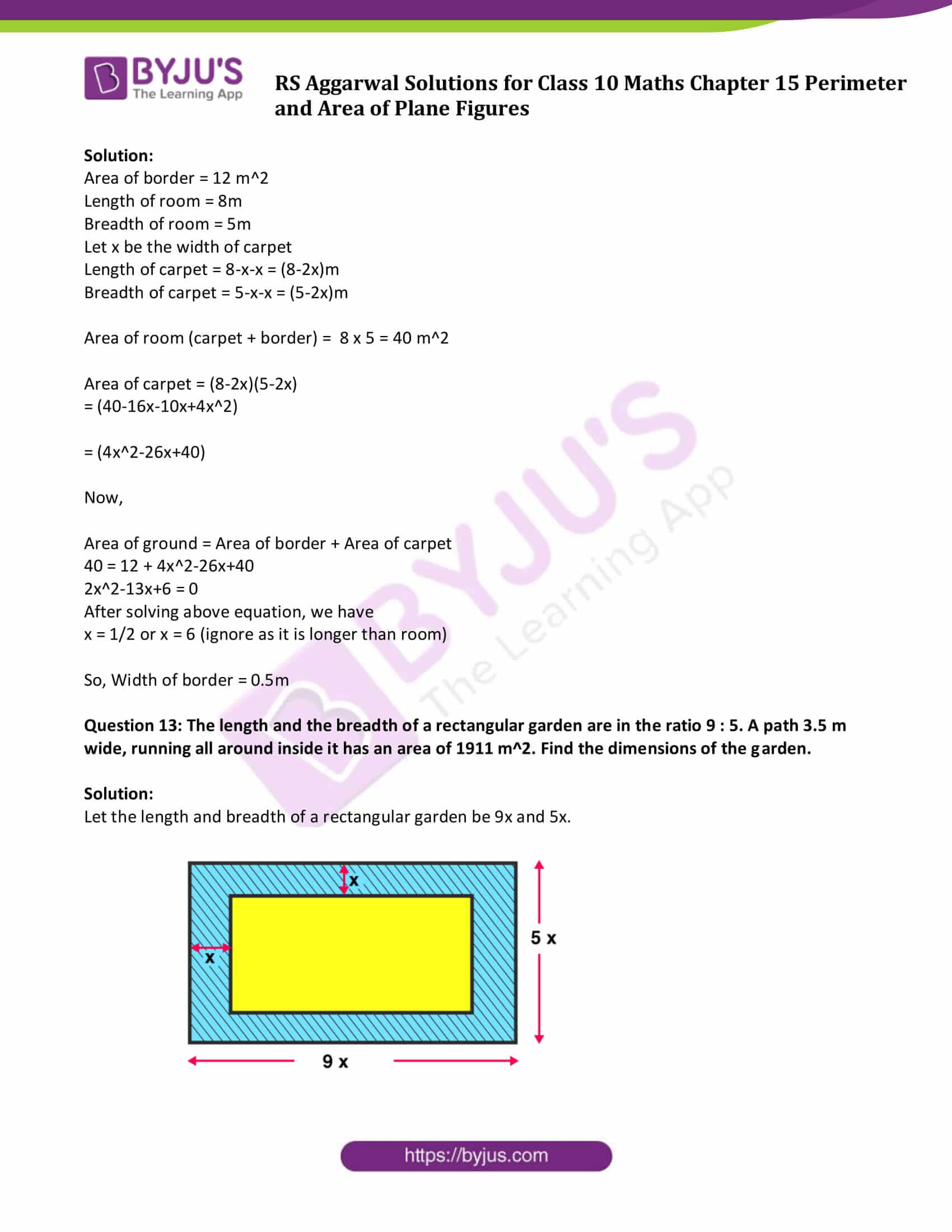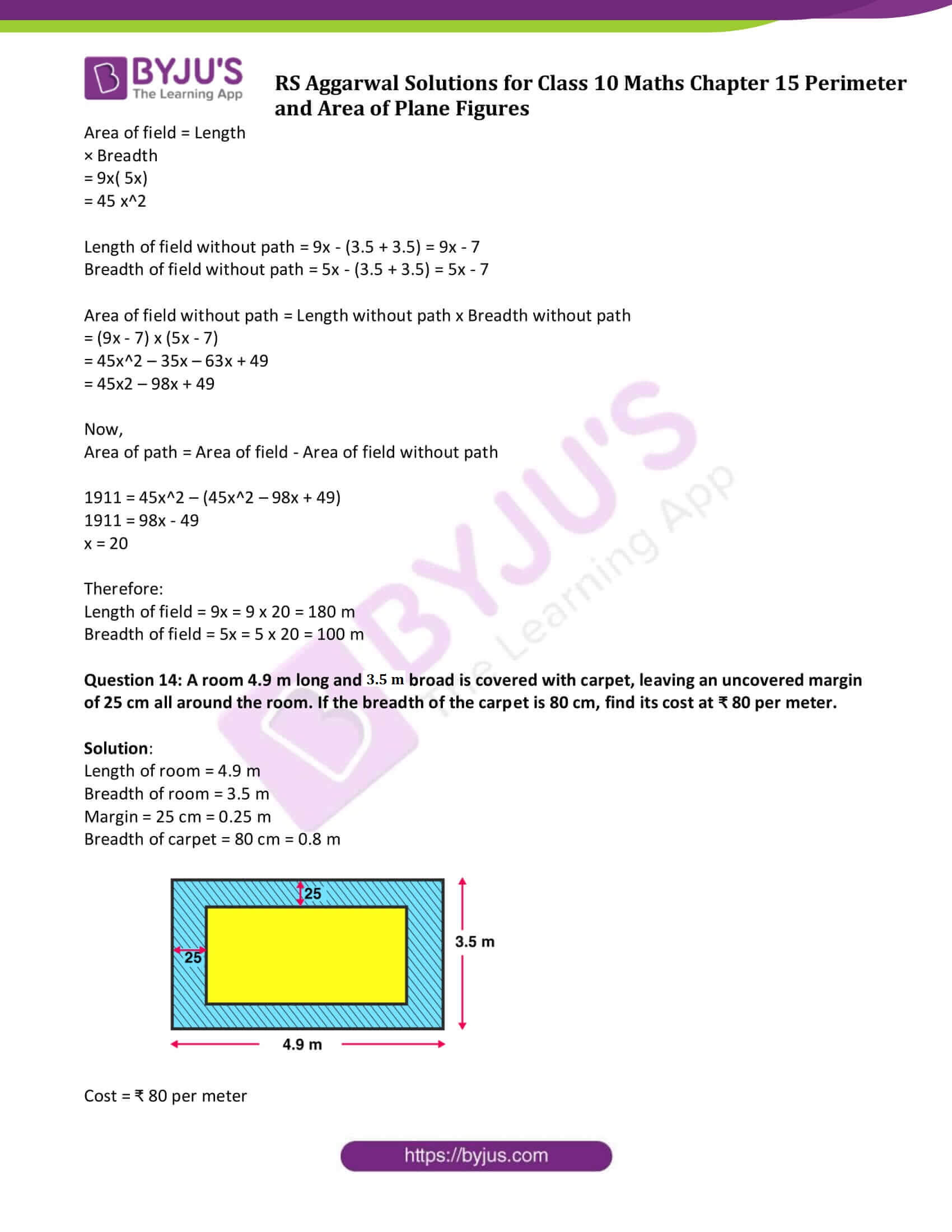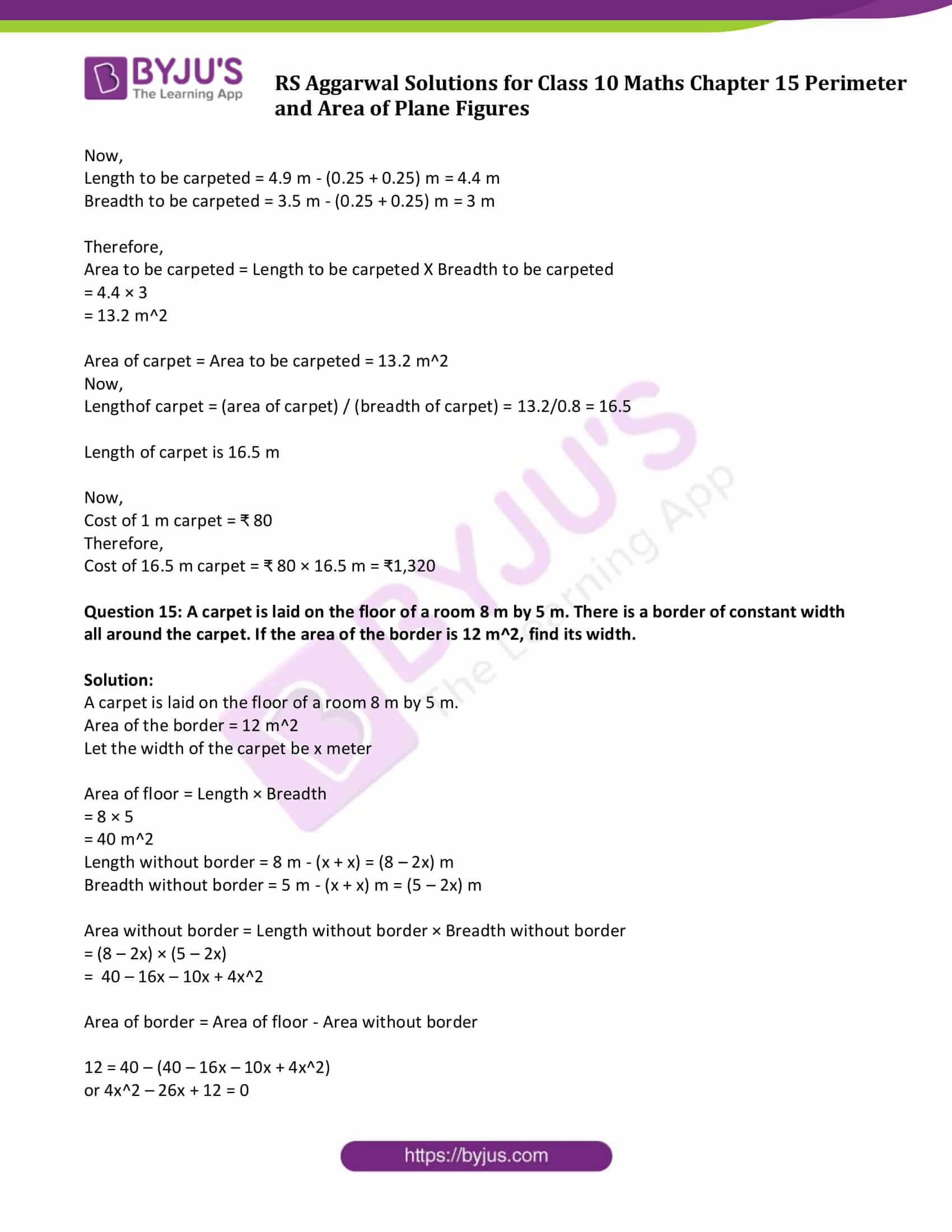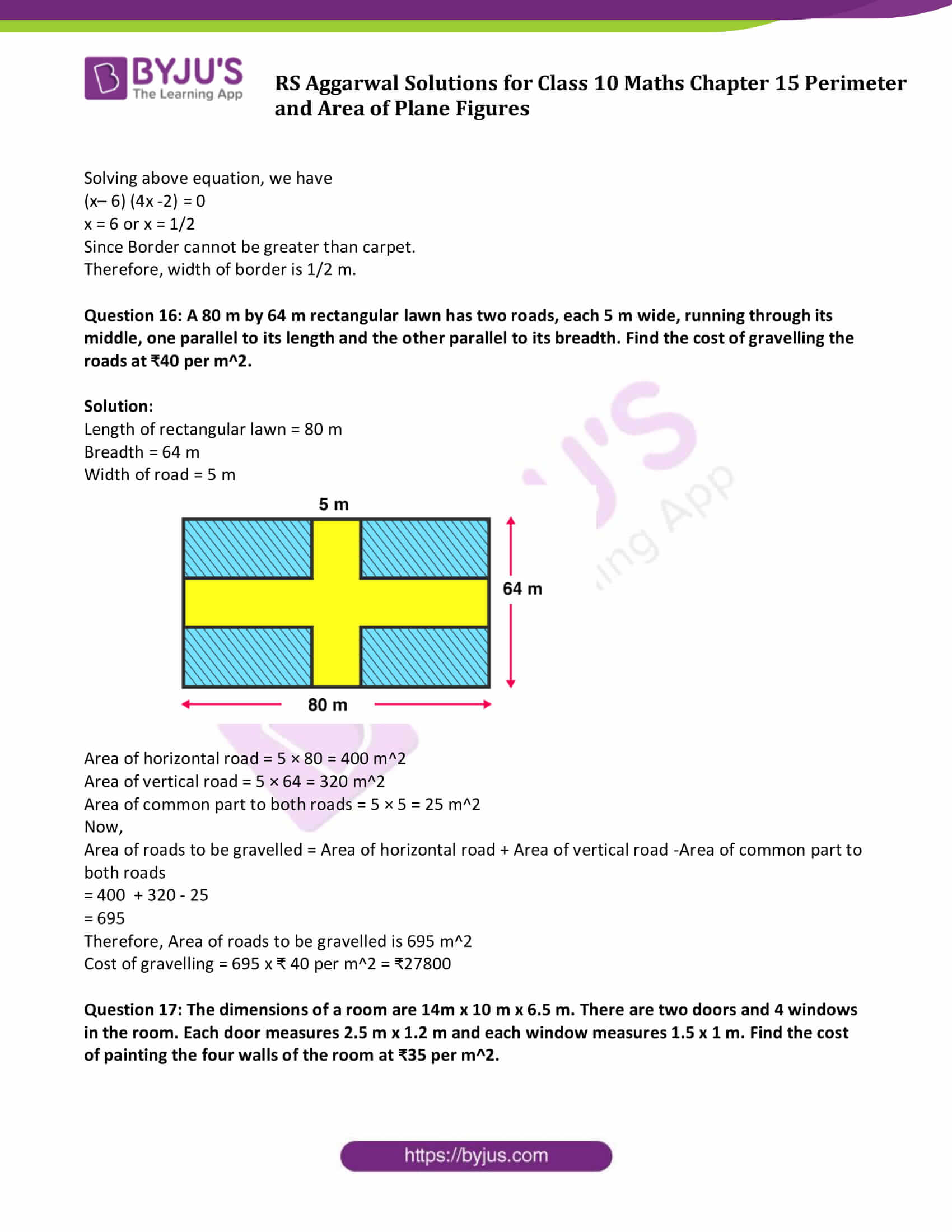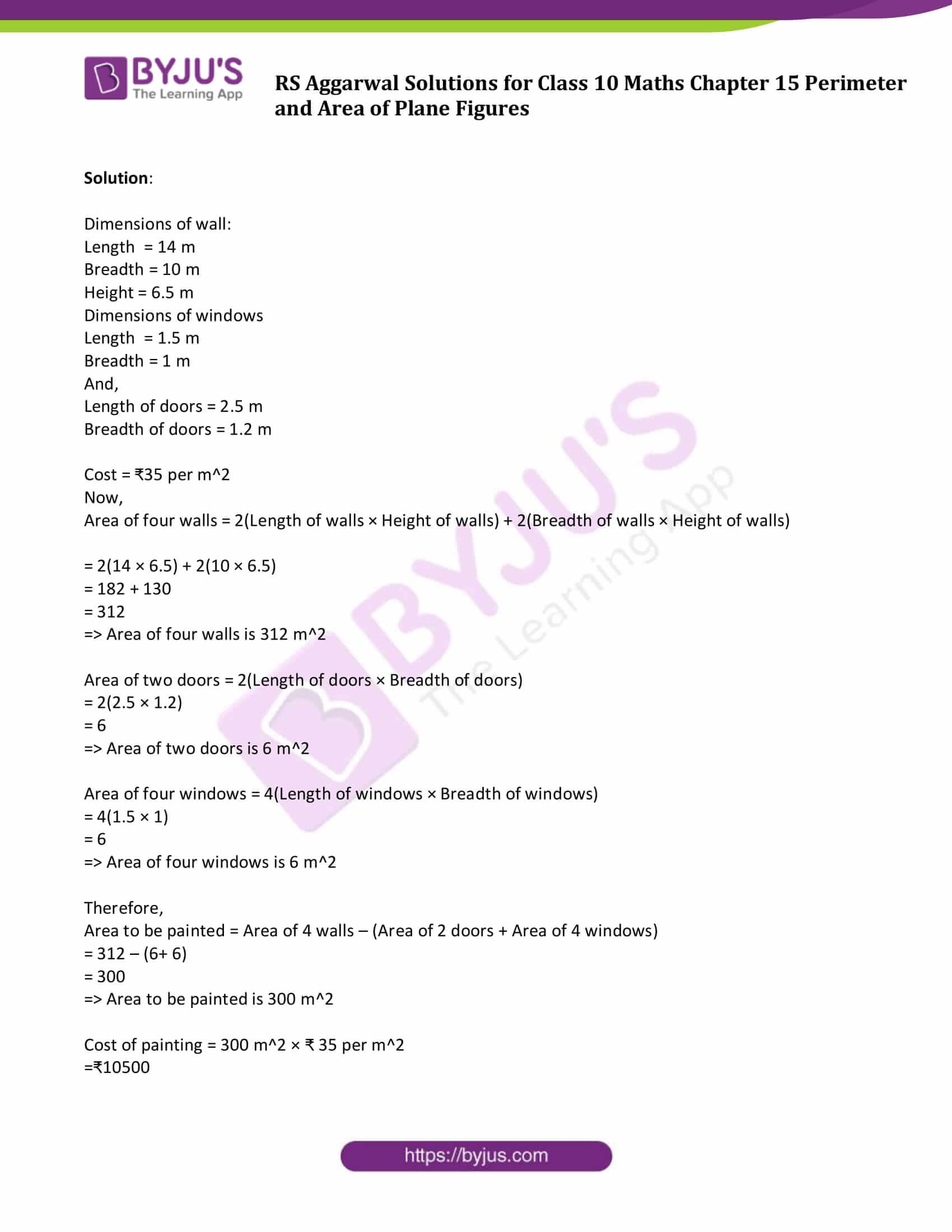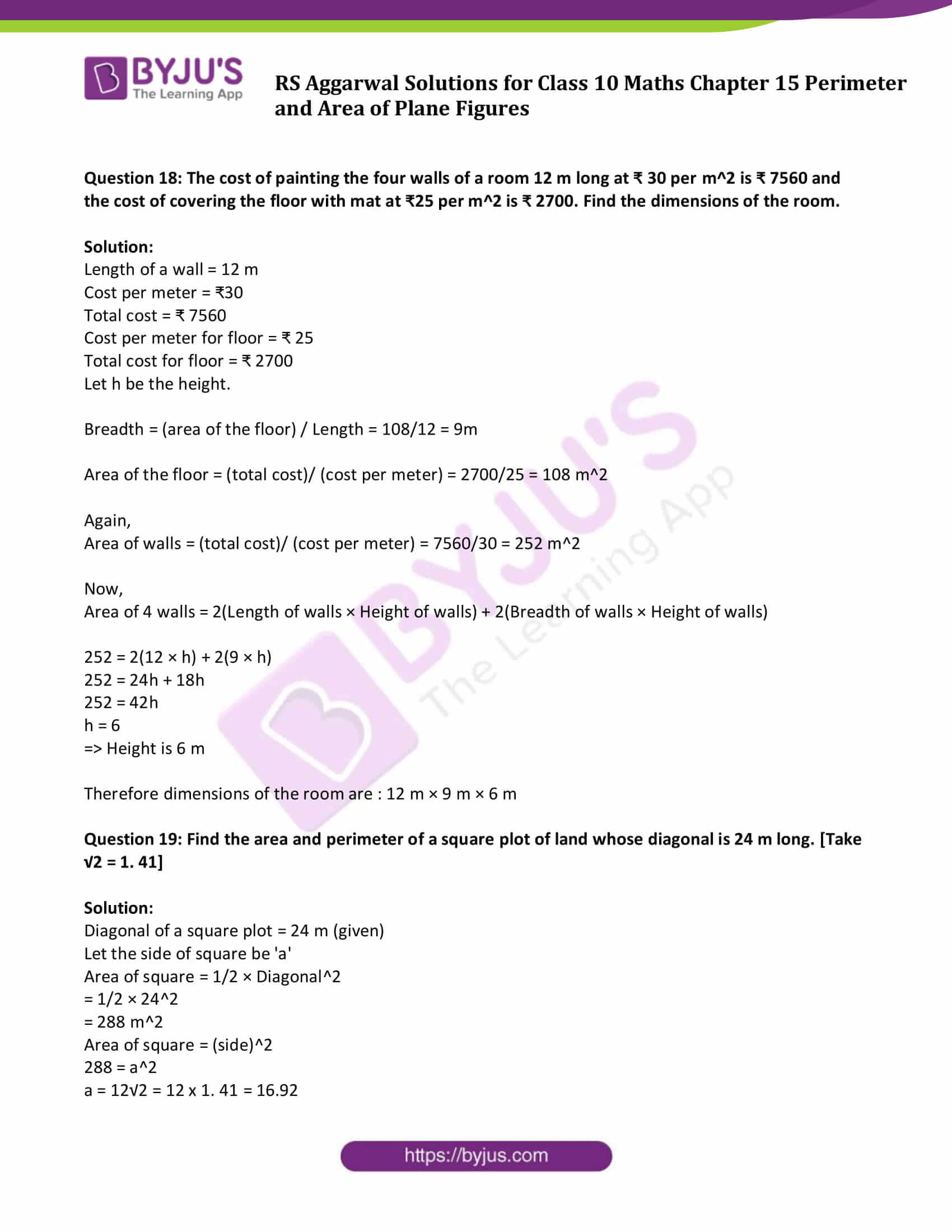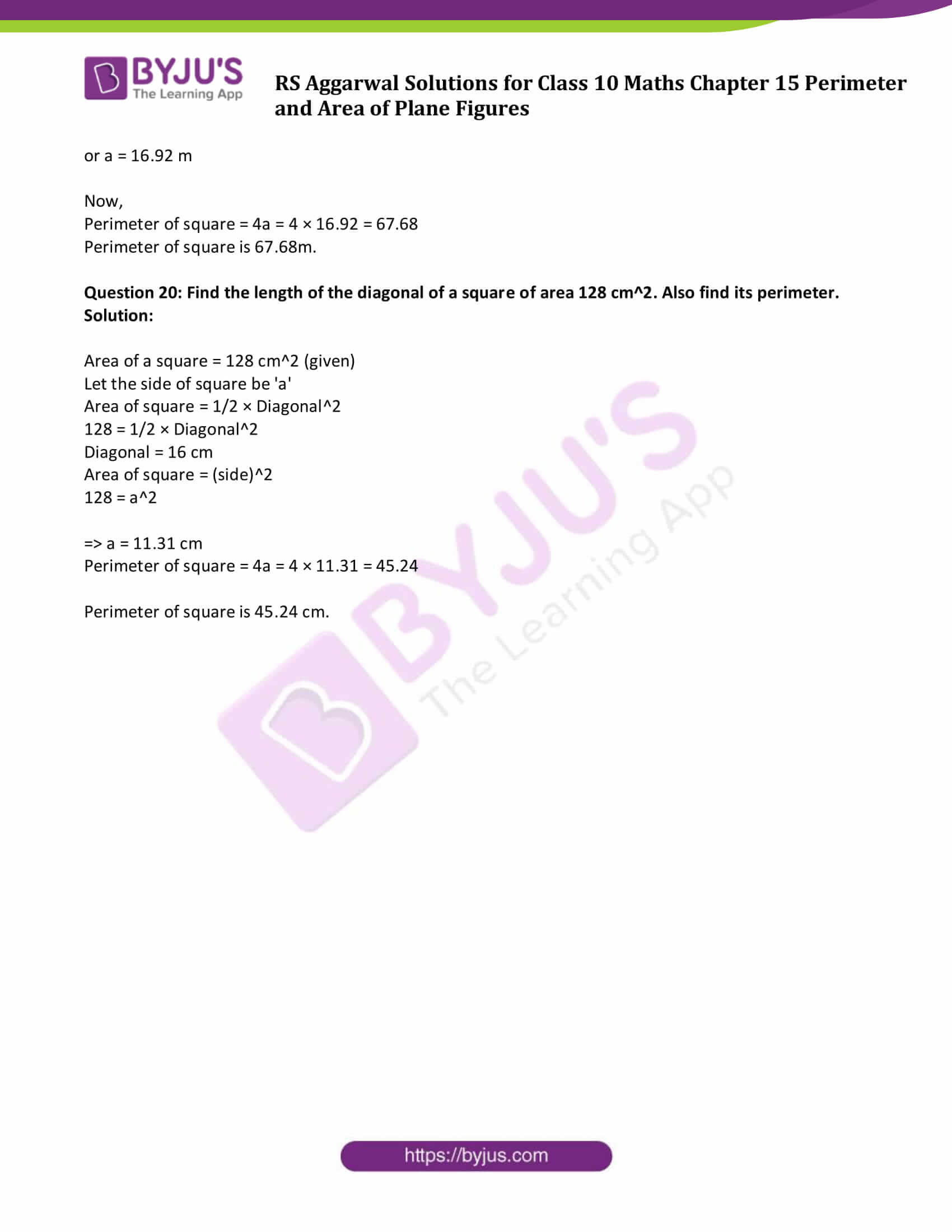### Access other exercise solutions of Class 10 Maths Chapter 15 Perimeter and Area of Plane Figures

Exercise 15 A Solutions

## Exercise 15B

Question 1: The perimeter of a rectangular plot of land is 80 m and its breadth is 16 m. Find the length and area of the plot.

Solution:

Perimeter of rectangle = 80 m

Let x be the length and 16m breadth.

We know, perimeter = 2 [length + breadth]

75 = 2(x + 16)

75 = (2x + 32)

Or x = 21.5

Thus,

Length of the rectangle is 21. 5 meter

Again,

Area of the rectangular plot = Length X Breadth

= (16 × 21.5)

= 344

So, Area of the rectangular plot is 344 cm^2

Therefore, length of rectangle is 21.5 m and the area is 344 m^2.

Question 2: The length of a rectangular park is twice its breadth, and its perimeter measures 840 m. Find the area of the park.

Solution:

The length of a rectangular park = 2(its breadth)

Perimeter = 840 m (Given)

Let x be the breadth of a rectangular park then, length of a rectangular park is 2x m.

We know, Perimeter of rectangle = 2(Length + Breadth)

840 = 2(2x + x)

840 = 6x

or x = 140

Breadth = x = 140 m

and Length = 2x = 2 x 140 = 280 m

Area of a rectangular park = Length x Breadth = 140 x 280 = 39200 m^2.

Question 3: One side of a rectangle is 12 cm long and its diagonal measures 37 cm. Find the other side and the area of the rectangle.

Solution:

Let ABCD be the rectangle in which AB

Let length of rectangle = 12 cm and diagonal= 37 m

Let breadth be the other side.

By Pythagoras theorem,

= v(49 x 25)

= v(1225)

Thus, length = 12 cm and breadth = 35 cm

Area of rectangle = (12 × 35) = 420

Hence, the other side is 35 cm and the area of rectangle is 420 cm^2.

Question 4: The area of a rectangular plot is 462 m^2 and its length is 28 m. Find the perimeter of the plot.

Solution:

Area of a rectangular plot = 462 m^2

Length of rectangle = 28 m

Let x m be the breadth of the plot

Now,

Area = Length × Breadth = (28x)

462 = 28x

or x = 16.5

Again,

Perimeter of the plot = 2(length + breadth) = 2(28 + 16.5) = 89

Therefore,

Breadth of plot = 16. 5 m

Perimeter of the plot = 89 m

Question 5: A lawn is in the form of a rectangle whose sides are in the ratio 5 : 3. The area of the lawn is 3375 m^2. Find the cost of fencing the lawn at ₹65 per metre.

Solution:

Cost of fencing lawn = ₹ 65 per metre.

Area of lawn = 3375 m^2

Length = 5x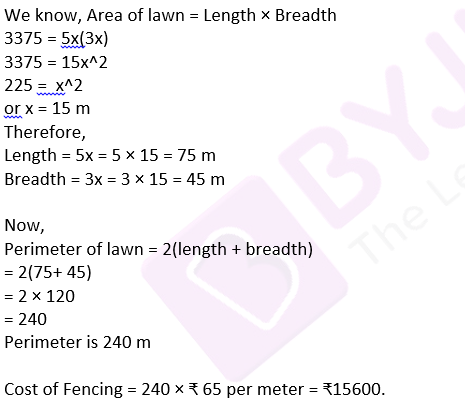Question 6: A room is 16 m long and 13.5 m broad. Find the cost of covering its floor with 75-cm-wide carpet at ₹60 per metre.

Solution:

Cost of covering room floor = ₹60 per metre.

Length of room = 16 m

Breadth of room = 13.5 m

Breadth of carpet = 75 cm = 0.75 m

We know, Area of room = Length × Breadth

= 16 × 13.5

= 216

⇨ Area of room is 216 m^2

Length of carpet can be calculated by using below formula:

Length of carpet = (area of room)/(Breadth of carpet)

= 216/0.75

= 288 m

Now,

Cost of covering the floor = 288 m × ₹60 per meter = ₹17280

Question 7: The floor of a rectangular hall is 24 m long and 18 m wide. How many carpets, each of length 2.5 m and breadth 80 cm, will be required to cover the floor of the hall?

Solution:

Length of hall = 24 m

Breadth of hall = 18 m

Length of carpet = 2.5 m

Breadth of carpet = 80 cm = 0.8 m

Area of hall = Length × Breadth

= 24 × 18

= 432 m^2

And, Area of carpet = Length × Breadth

= 2.5 × 0.8

= 2 m^2

Number of carpets = (area of hall)/(Area of carpet) = 432/2 = 216

That is, number of carpets are 216.

Question 8: A 36 m-long, 15m-broad verandah is to be paved with stones, each measuring 6 dm by 5 dm. How many stones will be required?

Solution:

Length of verandah = 36 m

Breadth of verandah = 15 m

Length of stones = 6 dm = 0.6 m

Breadth of stones = 5 dm = 0.5 m

Now,

Area of verandah = Length × Breadth

= 36 x 15

= 540

Area = 540 m^2

Area of stones = Length × Breadth

= 0.6× 0.5

= 0.3

Area of stone = 0.3 m^2

Number of stones = (area of verandah)/(Area of stones) = 540/0.3 = 1800

That is, number of stones required are 1800.

Question 9: The area of a rectangle is 192 cm^2 and its perimeter is 56 cm. Find the dimensions of the rectangle.

Solution:

The area of a rectangle is 192 cm^2 and its perimeter is 56 cm.

let l cm be the length and b cm be the breadth.

Area of rectangle = Length × Breadth

192 = l × b

⇨ l = 192/b ….(1)

Perimeter of rectangle = 2(length + breadth)

56 = 2(l + b)

from (1)

56 = 2(192/b + b)

28 = (192 + b^2)/b

b^2- 28b + 192 = 0

(b – 12 )(b – 16 ) = 0

b = 12 cm or b = 16 cm

Choose b = 12cm then l = 16 cm

Hence, Length is 16 cm and Breadth is 12 cm.

Question 10: A rectangular park 35 m long 18 m wide is to be covered with grass, leaving 2.5 m uncovered all around it. Find the area to be laid with grass.

Solution:

Given:

Length of the park = 35 m

Breadth of the park = 18 m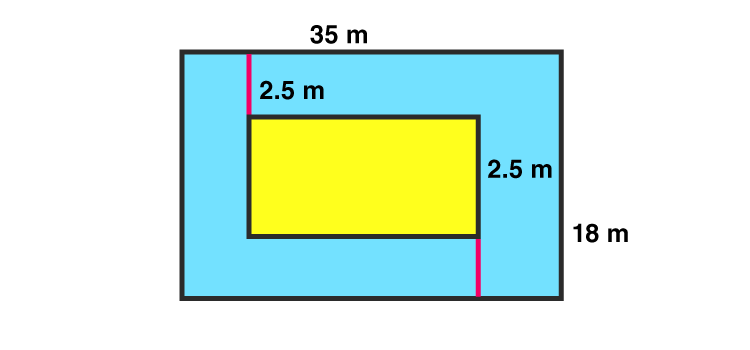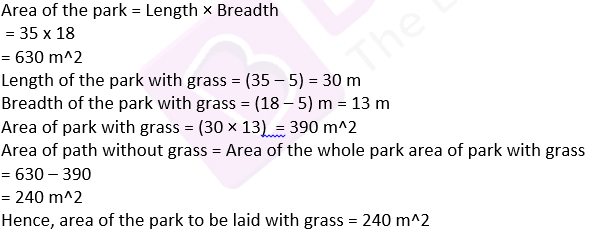Question 11: A rectangular plot measures 125m by 78m. It has gravel path 3m wide all around on the outside. Find the area of the path and the cost of gravelling it at ₹ 75 per m^2.

Solution:

Given:

Length of the plot = 125 m

Breadth of the plot = 78 m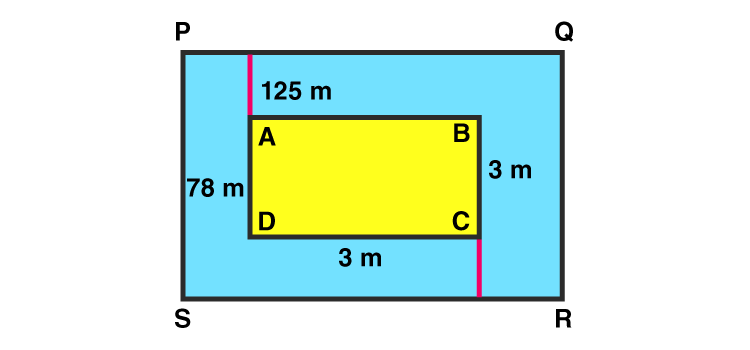Area of plot ABCD = Length × Breadth

= 125 x 78

= 9750 m^2

Length of the plot including the path= (125 + 3 + 3) m = 131 m

Breadth of the plot including the path = (78 + 3 + 3) m = 84 m

Now,

Area of plot PQRS including the path = Length × Breadth

= (131 × 84)

= 11004 m^2

Area of path = Area of plot PQRS – Area of plot ABCD

= 11004 – 9750

= 1254 m^2

Cost of gravelling = ₹75 per m^2

Cost of gravelling the whole path = ₹ (1254 × 75) = ₹ 94050

Hence, cost of gravelling the path is ₹ 94050.

Question 12: (i) A footpath of uniform width runs all around the inside of a rectangular field 54 m long and 35m wide. If the area of the path is 420 m^2, find the width of the path.

Solution:

Given:

Length of field = 54 m

Breadth of field = 35 m

Let x m be the width of the path.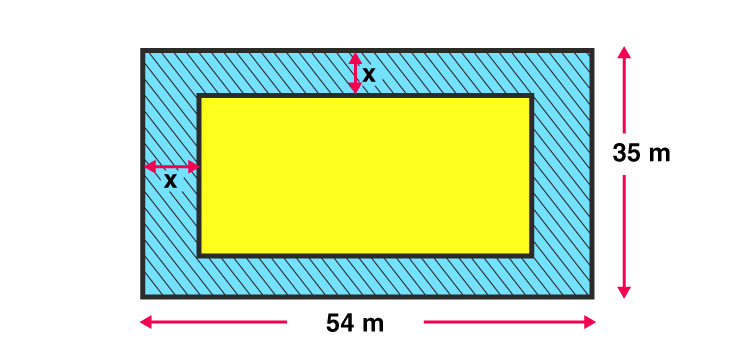Area of field = Length × Breadth

= 54 x 35

= 1890 m^2

Length of field without path = 54 – (x + x) = 54 – 2x

Breadth of field without path = 35 – (x + x) = 35 – 2x

Now,

Area of field without path = Length without path × Breadth without path

= (54 – 2x)(35 – 2x)

= 1890 – 70x – 108x + 4x^2

= 4x^2 – 178x + 1890 …(1)

Area of path = Area of field – Area of field without path

420 = 1890 – (1890 – 178x + 4x^2)

(Using equation (1))

420 = 178x – 4x^2

or 4x^2 – 178x + 420 = 0

or 2x^2 – 89x + 210 = 0

On solving above equation, we have

x = 42 or x = 5/2 m = 2.5 m

As width of park cannot be more than breadth of field, so width of park is 2.5 m.

(ii) A carpet is laid on the floor of a room 8m by 5m. There is a border of constant width all around the carpet. If the area of the border is 12 m^2, find its width.

Solution:

Area of border = 12 m^2

Length of room = 8m

Let x be the width of carpet

Length of carpet = 8-x-x = (8-2x)m

Breadth of carpet = 5-x-x = (5-2x)m

Area of room (carpet + border) = 8 x 5 = 40 m^2

Area of carpet = (8-2x)(5-2x)

= (40-16x-10x+4x^2)

= (4x^2-26x+40)

Now,

Area of ground = Area of border + Area of carpet

40 = 12 + 4x^2-26x+40

2x^2-13x+6 = 0

After solving above equation, we have

x = 1/2 or x = 6 (ignore as it is longer than room)

So, Width of border = 0.5m

Question 13: The length and the breadth of a rectangular garden are in the ratio 9 : 5. A path 3.5 m wide, running all around inside it has an area of 1911 m^2. Find the dimensions of the garden.

Solution:

Let the length and breadth of a rectangular garden be 9x and 5x.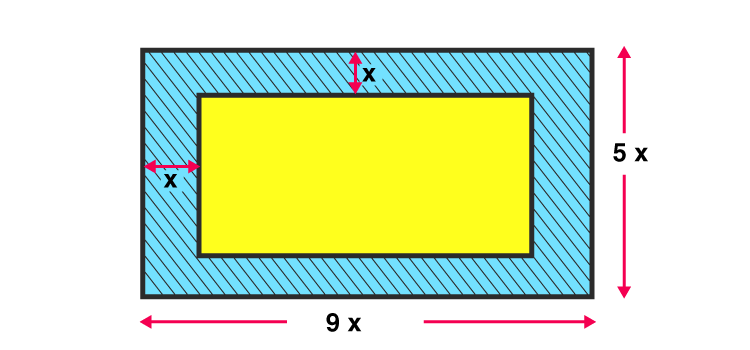Area of field = Length × Breadth

= 9x( 5x)

= 45 x^2

Length of field without path = 9x – (3.5 + 3.5) = 9x – 7

Breadth of field without path = 5x – (3.5 + 3.5) = 5x – 7

Area of field without path = Length without path x Breadth without path

= (9x – 7) x (5x – 7)

= 45x^2 – 35x – 63x + 49

= 45×2 – 98x + 49

Now,

Area of path = Area of field – Area of field without path

1911 = 45x^2 – (45x^2 – 98x + 49)

1911 = 98x – 49

x = 20

Therefore:

Length of field = 9x = 9 x 20 = 180 m

Breadth of field = 5x = 5 x 20 = 100 m

Question 14: A room 4.9 m long and 3.5 m broad is covered with carpet, leaving an uncovered margin of 25 cm all around the room. If the breadth of the carpet is 80 cm, find its cost at ₹ 80 per meter.

Solution:

Length of room = 4.9 m

Breadth of room = 3.5 m

Margin = 25 cm = 0.25 m

Breadth of carpet = 80 cm = 0.8 m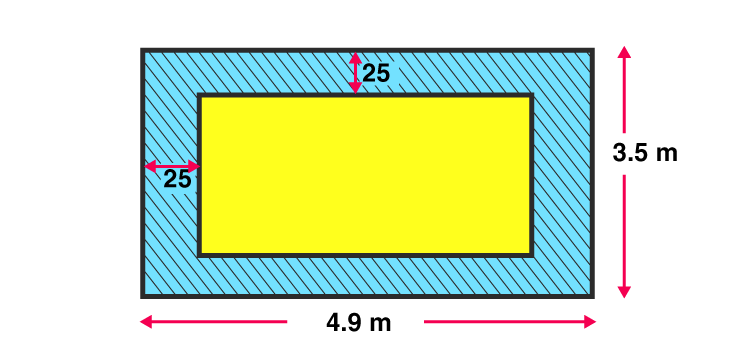Cost = ₹ 80 per meter

Now,

Length to be carpeted = 4.9 m – (0.25 + 0.25) m = 4.4 m

Breadth to be carpeted = 3.5 m – (0.25 + 0.25) m = 3 m

Therefore,

Area to be carpeted = Length to be carpeted X Breadth to be carpeted

= 4.4 × 3

= 13.2 m^2

Area of carpet = Area to be carpeted = 13.2 m^2

Now,

Lengthof carpet = (area of carpet) / (breadth of carpet) = 13.2/0.8 = 16.5

Length of carpet is 16.5 m

Now,

Cost of 1 m carpet = ₹ 80

Therefore,

Cost of 16.5 m carpet = ₹ 80 × 16.5 m = ₹1,320

Question 15: A carpet is laid on the floor of a room 8 m by 5 m. There is a border of constant width all around the carpet. If the area of the border is 12 m^2, find its width.

Solution:

A carpet is laid on the floor of a room 8 m by 5 m.

Area of the border = 12 m^2

Let the width of the carpet be x meter

Area of floor = Length × Breadth

= 8 × 5

= 40 m^2

Length without border = 8 m – (x + x) = (8 – 2x) m

Breadth without border = 5 m – (x + x) m = (5 – 2x) m

Area without border = Length without border × Breadth without border

= (8 – 2x) × (5 – 2x)

= 40 – 16x – 10x + 4x^2

Area of border = Area of floor – Area without border

12 = 40 – (40 – 16x – 10x + 4x^2)

or 4x^2 – 26x + 12 = 0

Solving above equation, we have

(x– 6) (4x -2) = 0

x = 6 or x = 1/2

Since Border cannot be greater than carpet.

Therefore, width of border is 1/2 m.

Question 16: A 80 m by 64 m rectangular lawn has two roads, each 5 m wide, running through its middle, one parallel to its length and the other parallel to its breadth. Find the cost of gravelling the roads at ₹40 per m^2.

Solution:

Length of rectangular lawn = 80 m

Width of road = 5 m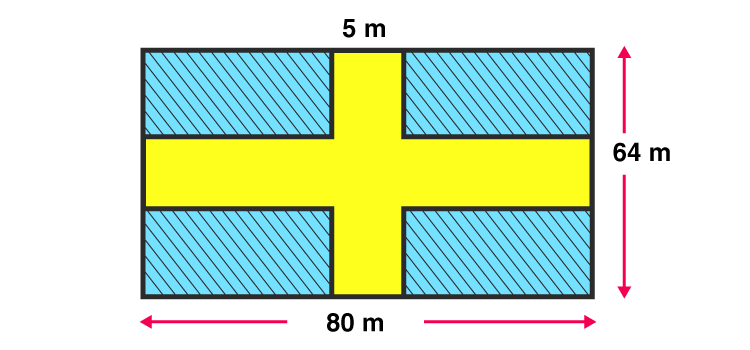Area of horizontal road = 5 × 80 = 400 m^2

Area of vertical road = 5 × 64 = 320 m^2

Area of common part to both roads = 5 × 5 = 25 m^2

Now,

Area of roads to be gravelled = Area of horizontal road + Area of vertical road -Area of common part to both roads

= 400 + 320 – 25

= 695

Therefore, Area of roads to be gravelled is 695 m^2

Cost of gravelling = 695 x ₹ 40 per m^2 = ₹27800

Question 17: The dimensions of a room are 14m x 10 m x 6.5 m. There are two doors and 4 windows in the room. Each door measures 2.5 m x 1.2 m and each window measures 1.5 x 1 m. Find the cost of painting the four walls of the room at ₹35 per m^2.

Solution:

Dimensions of wall:

Length = 14 m

Height = 6.5 m

Dimensions of windows

Length = 1.5 m

And,

Length of doors = 2.5 m

Breadth of doors = 1.2 m

Cost = ₹35 per m^2

Now,

Area of four walls = 2(Length of walls × Height of walls) + 2(Breadth of walls × Height of walls)

= 2(14 × 6.5) + 2(10 × 6.5)

= 182 + 130

= 312

⇨ Area of four walls is 312 m^2

Area of two doors = 2(Length of doors × Breadth of doors)

= 2(2.5 × 1.2)

= 6

⇨ Area of two doors is 6 m^2

Area of four windows = 4(Length of windows × Breadth of windows)

= 4(1.5 × 1)

= 6

⇨ Area of four windows is 6 m^2

Therefore,

Area to be painted = Area of 4 walls – (Area of 2 doors + Area of 4 windows)

= 312 – (6+ 6)

= 300

⇨ Area to be painted is 300 m^2

Cost of painting = 300 m^2 × ₹ 35 per m^2

=₹10500

Question 18: The cost of painting the four walls of a room 12 m long at ₹ 30 per m^2 is ₹ 7560 and the cost of covering the floor with mat at ₹25 per m^2 is ₹ 2700. Find the dimensions of the room.

Solution:

Length of a wall = 12 m

Cost per meter = ₹30

Total cost = ₹ 7560

Cost per meter for floor = ₹ 25

Total cost for floor = ₹ 2700

Let h be the height.

Breadth = (area of the floor) / Length = 108/12 = 9m

Area of the floor = (total cost)/ (cost per meter) = 2700/25 = 108 m^2

Again,

Area of walls = (total cost)/ (cost per meter) = 7560/30 = 252 m^2

Now,

Area of 4 walls = 2(Length of walls × Height of walls) + 2(Breadth of walls × Height of walls)

252 = 2(12 × h) + 2(9 × h)

252 = 24h + 18h

252 = 42h

h = 6

⇨ Height is 6 m

Therefore dimensions of the room are : 12 m × 9 m × 6 m

Question 19: Find the area and perimeter of a square plot of land whose diagonal is 24 m long. [Take √2 = 1. 41]

Solution:

Diagonal of a square plot = 24 m (given)

Let the side of square be ‘a’

Area of square = 1/2 × Diagonal^2

= 1/2 × 24^2

= 288 m^2

Area of square = (side)^2

288 = a^2

a = 12√2 = 12 x 1. 41 = 16.92

or a = 16.92 m

Now,

Perimeter of square = 4a = 4 × 16.92 = 67.68

Perimeter of square is 67.68m.

Question 20: Find the length of the diagonal of a square of area 128 cm^2. Also find its perimeter.

Solution:

Area of a square = 128 cm^2 (given)

Let the side of square be ‘a’

Area of square = 1/2 × Diagonal^2

128 = 1/2 × Diagonal^2

Diagonal = 16 cm

Area of square = (side)^2

128 = a^2

⇨ a = 11.31 cm

Perimeter of square = 4a = 4 × 11.31 = 45.24

Perimeter of square is 45.24 cm.

## R S Aggarwal Solutions for Class 10 Maths Chapter 15 Perimeter and Area of Plane Figures Exercise 15B

Class 10 Maths Chapter 15 Perimeter and Area of Plane Figures Exercise 15B is based on the area and perimeter of plane figures. In this exercise, students will be given real-life questions such as, if the length of a rectangular park is twice its breadth, and its perimeter measures 840 m. Find the area of the park. Such real-life based problems helpful to develop creative abilities in students. Students can download R S Aggarwal Solutions of Class 10 Chapter 15 to practice more questions.